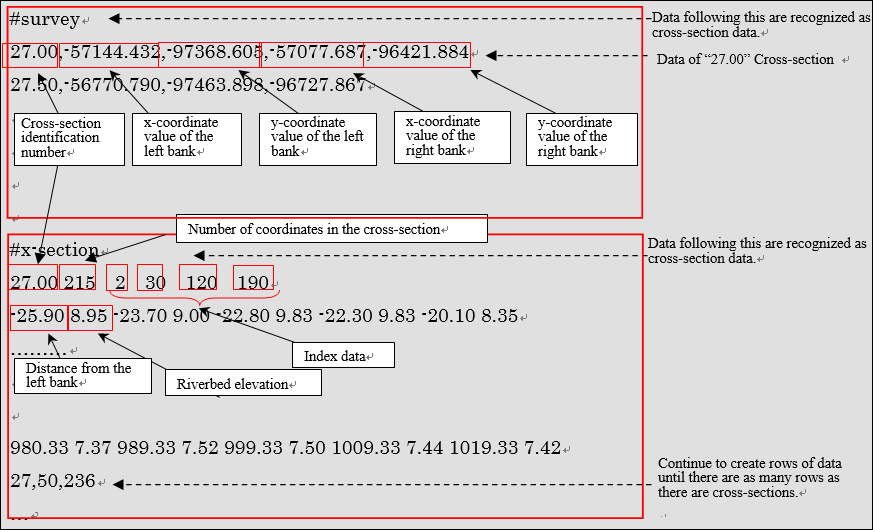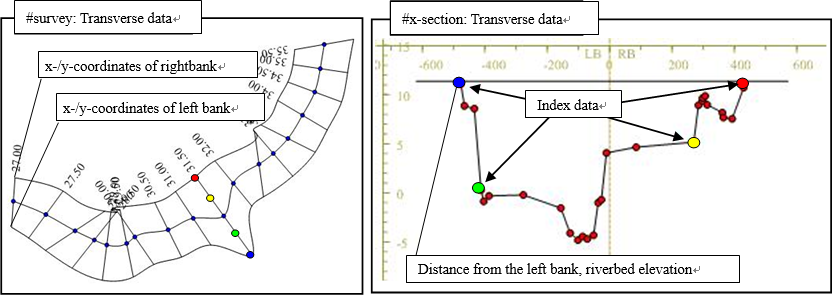# Cross-Section File (*.riv)¶

## Overview¶

The Cross-Section Data (*.riv) contains transverse data (x and y coordinates of left/right banks) and cross-section data (distance from left bank and riverbed elevation).

Cross-Section Data should be ASCII files. Figure 478 and Figure 6‑1 show the structure and concept, respectively.

• Break points of input data (for example, cross-section identification number and x-coordinate value) are identified by “space”, “tab” or “enter” characters. When break points are properly identified, iRIC automatically recognizes the data. Please note that cross-section identification number must be a real number.

• Rows after the “#survey” row are recognized as cross-section data.

• Each row contains data for one cross-section.
• Data row: (Cross-section identification number) (x-coordinate value of the left bank) (y-coordinate value of the left bank) (x-coordinate value of the right bank) (y-coordinate value of the right bank)
• Rows after the “#x-section” row are recognized as cross-section data (consisting of the “header line” and “data lines”).

• Header line: (Cross-section identification number) (No. of coordinates) (Index 1) (Index 2) (Index 3) (Index 4)

Indexes 1 to 4 specify points on a cross-sectional coordinate system by sequential integer numbers. (The top is 1.) Data prior to Index 1 and after Index 4 are discarded. The point specified by Index 1 is set as the left bank and the point specified by Index 4 is set as the right bank. The points specified by Indexes 2 and 3 are to be the division points (or nodes) of the automatically created grid. The Center Point of the river is set at the point midway between Indexes 2 and 3.

You can omit Index data. In such case, all the coordinate cross-section data are read and the first point of the coordinate cross-section data is set as the left bank and the last point is set as the right bank. The Center Point of the river is set at the mid-point between the left and right banks.

When Index data are not set for every cross-section data, the index data of all cross-sections are ignored.

• Data line: (Distance from left bank) (Riverbed elevation) Continue to create rows of data until there are as many rows as there are cross-sections.

Each row describes up to five sets of combinations of (Distance from left bank) and (Riverbed elevation) data.Figure 478 Structure of Cross-Section DataFigure 479 Concept of Cross-Section Data

Figure 479 shows the concept of the Cross-Section Data. iRIC does not display the four dots (data of Indexes 1 to 4).

The coordinates in the cross-sectional direction displayed in the [Cross-section] window have been converted as follows; note that they are different from [Distance from left bank] in the cross-section data of the Cross-Section Data.

• The coordinates of the Center Point of the river have been calculated from the longitudinal data and the cross-section data.
• The distance from the Center Point along the cross-sectional line has been calculated.

### CSV files for importing river center line¶

When importing Cross-Section Data, river center line can be imported from CSV files. Refer to Points CSV file (*.csv) for the file format.

## Scheduled driver longitudinal/cross-section data creation guideline and cross-sectional Cross-Section Data¶

The Ministry of Land, Infrastructure, Transport and Tourism () publishes guidelines for creating the scheduled river longitudinal/cross-section data

*http://www.mlit.go.jp/river/shishin_guideline/kasen/gis/pdf_docs/juoudan/guideline0805.pdf*

Table 44 shows how to convert the Guideline data items to the River Survey Data items.

Table 44 Relationship between the Cross-Section Data items and the Guideline data
Cross-Section Data item How to convert the guideline data (surveyed cross-sectional numerical data) to Cross-Section Data items
Coordinates of left/right banks Specify the coordinates of the left/right bank distance posts.
Cross-section data
Specify the distance of the cross-sectional coordinate data for the distance from the left bank.
Specify the elevation of the cross-sectional coordinate data.
Index data
Set as follows:
Index 1: Number that corresponds to the left bank distance post
Index 2: Number that corresponds to the left bank shoreline post
Index 3: Number that corresponds to the right bank shoreline post
Index 4: Number that corresponds to the right bank distance post